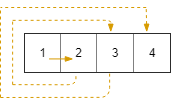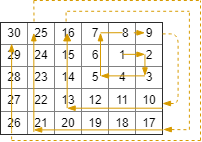## Algorithm

Problem Name: 885. Spiral Matrix III

You start at the cell `(rStart, cStart)` of an `rows x cols` grid facing east. The northwest corner is at the first row and column in the grid, and the southeast corner is at the last row and column.

You will walk in a clockwise spiral shape to visit every position in this grid. Whenever you move outside the grid's boundary, we continue our walk outside the grid (but may return to the grid boundary later.). Eventually, we reach all `rows * cols` spaces of the grid.

Return an array of coordinates representing the positions of the grid in the order you visited them.

Example 1:```Input: rows = 1, cols = 4, rStart = 0, cStart = 0
Output: [[0,0],[0,1],[0,2],[0,3]]
```

Example 2:```Input: rows = 5, cols = 6, rStart = 1, cStart = 4
Output: [[1,4],[1,5],[2,5],[2,4],[2,3],[1,3],[0,3],[0,4],[0,5],[3,5],[3,4],[3,3],[3,2],[2,2],[1,2],[0,2],[4,5],[4,4],[4,3],[4,2],[4,1],[3,1],[2,1],[1,1],[0,1],[4,0],[3,0],[2,0],[1,0],[0,0]]
```

Constraints:

• `1 <= rows, cols <= 100`
• `0 <= rStart < rows`
• `0 <= cStart < cols`

## Code Examples

### #1 Code Example with Python Programming

```Code - Python Programming```

``````
class Solution:
def spiralMatrixIII(self, R, C, r0, c0):
direct, res, n, l, ind = [(-1, 0), (0, 1), (1, 0), (0, -1)], [[r0, c0]], R * C, 1, 1
while len(res) < n:
for __ in range(2):
for _ in range(l):
r0 += direct[ind]
c0 += direct[ind]
if 0 <= r0 < R and 0 <= c0 < C:
res.append([r0, c0])
ind = (ind + 1) % 4
l += 1
return res
``````
Copy The Code &

Input

cmd
rows = 1, cols = 4, rStart = 0, cStart = 0

Output

cmd
[[0,0],[0,1],[0,2],[0,3]]

### #2 Code Example with C# Programming

```Code - C# Programming```

``````
namespace LeetCode
{
public class _0885_SpiralMatrixIII
{
public int[][] SpiralMatrixIII(int R, int C, int r0, int c0)
{
int[] dr = new int[] { 0, 1, 0, -1 };
int[] dc = new int[] { 1, 0, -1, 0 };

int[][] result = new int[R * C][];
int index = 0, direction = 0, step = 2;
result[index++] = new int[] { r0, c0 };

while (index < R * C)
{
var distance = step / 2;
for (int i = 0; i < distance; i++)
{
r0 += dr[direction];
c0 += dc[direction];

if (r0 >= 0 && r0 < R && c0 >= 0 && c0 < C)
{
result[index++] = new int[] { r0, c0 };
if (index == R * C) break;
}
}

direction++;
direction %= 4;
step++;
}

return result;
}
}
}
``````
Copy The Code &

Input

cmd
rows = 1, cols = 4, rStart = 0, cStart = 0

Output

cmd
[[0,0],[0,1],[0,2],[0,3]]